# Calculating the First Tuesdayby Allen Wyatt
(last updated June 16, 2018)

Christine needs a way to calculate the first Tuesday of any given month. So, given a month and a year, she needs to get the date of that first Tuesday.

There are quite a few different formulas you can use to achieve the desired result. Most approaches use the WEEKDAY function in some way because it can return a numeric value representing the day of the week for any given date. Assuming that you put a month in cell A1 (1 through 12) and a four-digit year in cell A2, then the following formulas will all return a date for the first Tuesday in the month. (I've arranged the formulas in order of length, from longest to shortest.)

```=DATE(A2,A1,1)+IF(WEEKDAY(DATE(A2,A1,1))=3,0,IF(WEEKDAY(DATE(A2,A1,1))>3,7-WEEKDAY(DATE(A2,A1,1))+3,3-WEEKDAY(DATE(A2,A1,1))))
=IF(3-WEEKDAY(DATE(A2,A1,1))>=0,DATE(A2,A1,1)+3-WEEKDAY(DATE(A2,A1,1)),DATE(A2,A1,1)+7+3-WEEKDAY(DATE(A2,A1,1)))
=DATE(A2,A1,1)+IF(3-WEEKDAY(DATE(A2,A1,1))>=0,3-WEEKDAY(DATE(A2,A1,1)),10-WEEKDAY(DATE(A2,A1,1)))
=DATE(A2,A1,1)-WEEKDAY(DATE(A2,A1,1),1)+3+IF(WEEKDAY(DATE(A2,A1,1),1)>3,7,0)
=DATE(A2,A1,1)+8-DAY(DATE(A2,A1,1))-WEEKDAY(DATE(A2,A1,1)-DAY(DATE(A2,A1,1))-2)
=DATE(A2,A1,1)+3-WEEKDAY(DATE(A2,A1,1))+(1-(3>=WEEKDAY(DATE(A2,A1,1))))*7
=DATEVALUE(A2&"/"&A1&"/1")+MOD(10-WEEKDAY(DATEVALUE(A2&"/"&A1&"/1")),7)
=DATE(A2,A1,MATCH(TRUE,WEEKDAY(DATE(A2,A1,{1,2,3,4,5,6,7}))=3,0))
=DATE(A2,A1,MOD(10-WEEKDAY(DATE(A2,A1,1)),7)+1)
=DATE(A2,A1,1)+MOD(3-WEEKDAY(DATE(A2,A1,1)),7)
=DATE(A2,A1,1)+7-WEEKDAY(DATE(A2,A1,1),13)
=DATE(A2,A1,8-WEEKDAY(DATE(A2,A1,1),13))
=DATE(A2,A1,7)-MOD(DATE(A2,A1,7)-3,7)
=DATE(A2,A1,8-WEEKDAY(DATE(A2,A1,5)))
=DATE(A2,A1,8)-WEEKDAY(DATE(A2,A1,5))
```

If you find it more convenient to specify a date within the month you want to evaluate, then you can modify the formulas to take that into account. The follow are just a few formulas you could use if you put an actual date into cell A1:

```=DATE(YEAR(A1),MONTH(A1),MATCH(TRUE,WEEKDAY(DATE(YEAR(A1),MONTH(A1),{1,2,3,4,5,6,7}))=3,0))
=DATE(YEAR(A1),MONTH(A1),MOD(10-WEEKDAY(DATE(YEAR(A1),MONTH(A1),1)),7)+1)
=DATE(YEAR(A1),MONTH(A1),1)+MOD(3-WEEKDAY(DATE(YEAR(A1),MONTH(A1),1)),7)
=DATE(YEAR(A1),MONTH(A1),1)+7-WEEKDAY(DATE(YEAR(A1),MONTH(A1),1),13)
=DATE(YEAR(A1),MONTH(A1),8-WEEKDAY(DATE(YEAR(A1),MONTH(A1),1),13))
=DATE(YEAR(A1),MONTH(A1),7)-MOD(DATE(YEAR(A1),MONTH(A1),7)-3,7)
=DATE(YEAR(A1),MONTH(A1),8-WEEKDAY(DATE(YEAR(A1),MONTH(A1),5)))
=DATE(YEAR(A1),MONTH(A1),8)-WEEKDAY(DATE(YEAR(A1),MONTH(A1),5))
=A1-DAY(A1)+7-WEEKDAY(A1-DAY(A1)-1,3)
```

Finally, you may want to consider a macro-based approach. The following is a user-defined function that quickly figures out the first Tuesday:

```Function FirstTuesday(M As Integer, Y As Integer) As Date
Dim dResult As Date

dResult = DateSerial(Y, M, 1)
Do While Weekday(dResult) <> vbTuesday
Loop
FirstTuesday = dResult
End Function
```

All you need to do in your worksheet is to pass the user-defined function the numeric month and year desired:

```=FirstTuesday(6,2018)
```

If you prefer, you can specify that the month and year are in a cell:

```=FirstTuesday(A2,A1)
```

ExcelTips is your source for cost-effective Microsoft Excel training. This tip (13540) applies to Microsoft Excel 2007, 2010, 2013, 2016, 2019, and Excel in Office 365.

##### Author Bio

Allen Wyatt

With more than 50 non-fiction books and numerous magazine articles to his credit, Allen Wyatt is an internationally recognized author. He is president of Sharon Parq Associates, a computer and publishing services company. ...

##### MORE FROM ALLEN

Do you find that there is a lot of extra space around the data on your worksheet when it is printed? Changing the margins ...

Discover More

Last Document Saves Not Saved

Click the Save button and you expect your document to be saved, right? What if you later discover it wasn't really saved? ...

Discover More

Changing Text in Text Boxes on a Chart

Macros allow you to make changes to virtually anything you can see in Excel. This tip examines how to make changes (even ...

Discover MoreProfessional Development Guidance! Four world-class developers offer start-to-finish guidance for building powerful, robust, and secure applications with Excel. The authors show how to consistently make the right design decisions and make the most of Excel's powerful features. Check out Professional Excel Development today!

##### More ExcelTips (ribbon)

Entering Dates without Separators

When doing data entry into a worksheet, you might want to enter dates without the need to type the separators that are ...

Discover More

Leap Years and Fiscal Periods

Need to figure out when a fiscal year ends when that period does not correspond to the calendar year? Here are some ways ...

Discover More

Pushing Dates Into Last Month

Excel is great when it comes to working with dates and times. You can even do math on dates. One such easy manipulation ...

Discover More
##### Subscribe

FREE SERVICE: Get tips like this every week in ExcelTips, a free productivity newsletter. Enter your address and click "Subscribe."

If you would like to add an image to your comment (not an avatar, but an image to help in making the point of your comment), include the characters [{fig}] in your comment text. You’ll be prompted to upload your image when you submit the comment. Maximum image size is 6Mpixels. Images larger than 600px wide or 1000px tall will be reduced. Up to three images may be included in a comment. All images are subject to review. Commenting privileges may be curtailed if inappropriate images are posted.

What is six minus 1?

2018-06-25 10:15:43

Willy Vanhaelen

@Peter
I think it's an execellent idea. It makes the formula easier to use. And it appropriate you can still use ± n days.

2018-06-25 09:43:12

Peter Atherton

Willy

Thanks for the new function and yes it is easier to understand. I will view Nick Rothstein and your comments after posting this.

I took the liberty of adding another variable to the Firstday function that I think makes it less likely to enter an error. What do you think?

Function FirstDay(Y As Integer, M As Integer, D As Integer, _
Optional wk2Add As Integer = 0) As Date
'Willy Vanhaelen
FirstDay = Application.Floor(DateSerial(Y, M, 7 - D), 7) + D + 7 * wk2Add
End Function

(see Figure 1 below)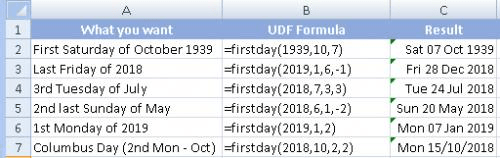Figure 1.

2018-06-24 12:58:55

Willy Vanhaelen

@Peter
Thanks for the compliment. While trying to answer your question I did some testing and realised that in this case the Evaluate method is not the best choice.
The result is an even shorter version of the UDF (and easier to understand):

Function FirstDay(Y As Integer, M As Integer, D As Integer) As Date
FirstDay = Application.Floor(DateSerial(Y, M, 7 - D), 7) + D
End Function

The Evaluate method is a good choice though to implement Excel's array formulas in VBA to avoid the use of a Loop resulting in much faster macros. If you are interested you can read the comments of Rick Rothstein and myself in this tip:
https://excelribbon.tips.net/T010768_Shortening_ZIP_Codes.html
It's a fact that that the Evaluate method is the most poorly commented item in Excel's Help. If you want more, simple Google for "vba evaluate".

BTW: there is an error in row 4 of my picture: Third Tuesday of July should be Fourth Tuesday (or +21 in the formula must by +14 to get the third).

2018-06-24 05:14:38

Peter Atherton

Willy

Excellent stuff as usual! The UDF is definitely one for the Personal workbook.

Can you point me to to a good write-up on the Evaluate function? Thanks.

2018-06-23 06:41:50

Willy Vanhaelen

Here is a one-liner User Defined Function that does it all:

Function FirstDay(Y As Integer, M As Integer, D As Integer) As Date
FirstDay = Evaluate("FLOOR(DATE(" & Y & "," & M & "," & 7 - D & "),7)+" & D)
End Function

Syntax: =FirstDay(year,month,weekday)
weekday: 1 for Sunday ... 7 for Saturday

If you need to use this often then place the macro in a module of your Personal workbook and it will be available in any workbook you open.
Here are some examples:

(see Figure 1 below)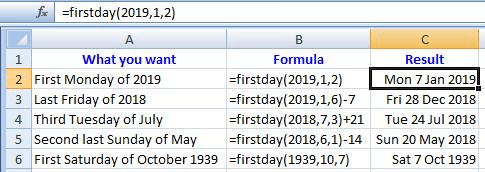Figure 1.

2018-06-20 14:38:58

Willy Vanhaelen

@Peter Atherton
Here is a much shorter formula to do the job:

=FLOOR(DATE(B2,B1,7-B4),7)+B4

(see Figure 1 below)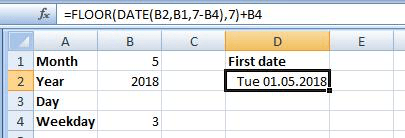Figure 1.

2018-06-20 09:19:40

Peter Atherton

Ignore the last post.

The following returns the first date for any workday.

=DATE(B2,B1,1)+ABS(7-WEEKDAY(DATE(B2,B1,1)+7-B4))

(see Figure 1 below)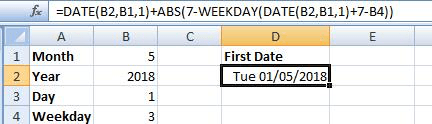Figure 1.

2018-06-19 06:31:05

Peter Atherton

Willy

a variation on your smashing formula

=CEILING(DATE(\$B\$2,\$B\$1,1+Rwkday),7)-(1+Rwkday) to find th first occurrunce

and
=(CEILING(DATE(\$B\$2,\$B\$1,1+Rwkday),7)-(1+Rwkday)+7*(WkNumber-1)) to get the nth occurence

(see Figure 1 below)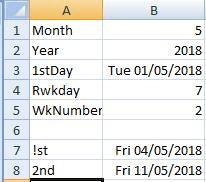Figure 1.

2018-06-17 08:08:38

Willy Vanhaelen

@Erik
If you use my formula you get the same result:
=CEILING(DATE(B2,B1,5),7)-4
but it is half the size :-).

2018-06-16 21:59:51

Erik

If you input the month and year of interest in two cells, one short formula will tell you the date of the first Tuesday of that month.
For example:
• input your month (1-12) in cell B1
• input the four-digit year in cell B2
• use this formula: =DATE(B2,B1,1)+7-WEEKDAY(DATE(B2,B1,1),13)
Be sure the formula cell is formatted as a date so the result is legible.

2018-06-16 08:28:37

Willy Vanhaelen

Here is probably the shortest possible formula for the first approuch:

=CEILING(DATE(A2,A1,5),7)-4

As for the macro, here is a one-liner:

Function FirstTuesday(M As Integer, Y As Integer) As Date
FirstTuesday = Evaluate("CEILING(DATE(" & Y & " ," & M & ",5),7)-4")
End Function

##### This Site

Got a version of Excel that uses the ribbon interface (Excel 2007 or later)? This site is for you! If you use an earlier version of Excel, visit our ExcelTips site focusing on the menu interface.